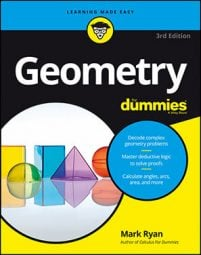##### Geometry For DummiesWhen you solve an analytic proof, this involves using algebra. You can use analytic proofs to prove different properties; for example, you can prove the property that the diagonals of a parallelogram bisect each other, or that the diagonals of an isosceles trapezoid are congruent.

Before solving a proof, it's useful to draw your figure in the coordinate system and label its vertices. Then you can concentrate on using algebra to prove something about the figure.

The following analytic proof walks you through this process: First, prove analytically that the midpoint of the hypotenuse of a right triangle is equidistant from the triangle's three vertices, and then show analytically that the median to this midpoint divides the triangle into two triangles of equal area.

After you complete your drawing, you're ready to do the algebraic part of the proof. The first part of the problem asks you to prove that the midpoint of the hypotenuse is equidistant from the triangle's vertices. To do that, start by determining the midpoint of the hypotenuse:

The following figure shows the midpoint, M, and median, line PM.

To prove the equidistance of M to P, Q, and R, you use the distance formula:

These distances are equal, and that completes the equidistance portion of the proof.

For the second part of the proof, you must show that the segment that goes from the right angle to the hypotenuse's midpoint divides the triangle into two triangles with equal areas—in other words, you have to show that

To compute these areas, you need to know the lengths of the base and altitude of both triangles. The next figure shows the triangles' altitudes.

Note that because base line PR of triangle PMR is horizontal, the altitude drawn to that base (line TM) is vertical, and thus you know that T is directly below (a, b) at (a, 0). With triangle PQM (using vertical base PQ), you create horizontal altitude SM and locate point S directly to the left of (a, b) at (0, b).

Now you're ready to use the two bases and two altitudes to show that the triangles have equal areas. To get the lengths of the bases and altitudes, you could use the distance formula, but you don't need to because you can use the following nifty shortcut for horizontal and vertical distances:

Time to wrap this up using the triangle area formula:

The areas are equal. That does it.